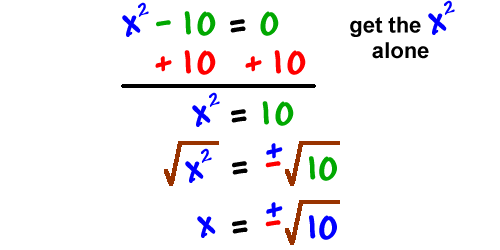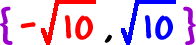Here's another one done both ways: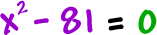WAY 1: Factoring WAY 2: Square root trick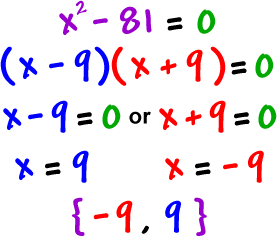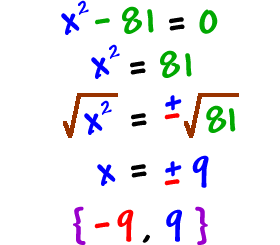Solve both ways:OK, so if we can just factor these things, why do we need the square root trick? Ah, because sometimes you can't factor them!

Check it out:

Solve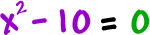Not the difference of two squares, is it?

So, we NEED the square root trick: